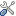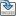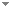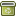# Dependence and Independence

Studia Logica 101 (2):399-410 (2013)

 Authors Jouko A Vaananen University of Helsinki Abstract We introduce an atomic formula ${\vec{y} \bot_{\vec{x}}\vec{z}}$ intuitively saying that the variables ${\vec{y}}$ are independent from the variables ${\vec{z}}$ if the variables ${\vec{x}}$ are kept constant. We contrast this with dependence logic ${\mathcal{D}}$ based on the atomic formula = ${(\vec{x}, \vec{y})}$ , actually equivalent to ${\vec{y} \bot_{\vec{x}}\vec{y}}$ , saying that the variables ${\vec{y}}$ are totally determined by the variables ${\vec{x}}$ . We show that ${\vec{y} \bot_{\vec{x}}\vec{z}}$ gives rise to a natural logic capable of formalizing basic intuitions about independence and dependence. We show that ${\vec{y} \bot_{\vec{x}}\vec{z}}$ can be used to give partially ordered quantifiers and IF-logic an alternative interpretation without some of the shortcomings related to so called signaling that interpretations using = ${(\vec{x}, \vec{y})}$ have Keywords Logics of dependence and independence  Team semantics  Logics with imperfectinformation  Axiomatization of independence Categories Logic and Philosophy of Logic (categorize this paper) ISBN(s) DOI 10.1007/s11225-013-9479-2 OptionsEdit this recordMark as duplicateExport citationFind it on ScholarRequest removal from indexRevision history

PhilArchive copy

Upload a copy of this paper     Check publisher's policy     Papers currently archived: 72,564

## References found in this work BETA

Compositional Semantics for a Language of Imperfect Information.W. Hodges - 1997 - Logic Journal of the IGPL 5 (4):539-563.
On Definability in Dependence Logic.Juha Kontinen & Jouko Väänänen - 2009 - Journal of Logic, Language and Information 18 (3):317-332.

## Citations of this work BETA

Questions and Dependency in Intuitionistic Logic.Ivano Ciardelli, Rosalie Iemhoff & Fan Yang - 2020 - Notre Dame Journal of Formal Logic 61 (1):75-115.
Propositional Logics of Dependence.Fan Yang & Jouko Väänänen - 2016 - Annals of Pure and Applied Logic 167 (7):557-589.
Generalized Quantifiers in Dependence Logic.Fredrik Engström - 2012 - Journal of Logic, Language and Information 21 (3):299-324.
Explicating Logical Independence.Lloyd Humberstone - 2020 - Journal of Philosophical Logic 49 (1):135-218.

## Similar books and articles

Generalized Quantifiers in Dependence Logic.Fredrik Engström - 2012 - Journal of Logic, Language and Information 21 (3):299-324.
From If to Bi.Samson Abramsky & Jouko Väänänen - 2009 - Synthese 167 (2):207 - 230.
On Definability in Dependence Logic.Juha Kontinen & Jouko Väänänen - 2009 - Journal of Logic, Language and Information 18 (3):317-332.
General Models and Entailment Semantics for Independence Logic.Pietro Galliani - 2013 - Notre Dame Journal of Formal Logic 54 (2):253-275.
Substance and Independence in Aristotle.Phil Corkum - 2013 - In B. Schnieder, A. Steinberg & M. Hoeltje (eds.), Varieties of Dependence: Ontological Dependence, Supervenience, and Response-Dependence. Basic Philosophical Concepts Series, Philosophia Verlag. pp. 36-67.
Logical Independence in Quantum Logic.Miklós Rédei - 1995 - Foundations of Physics 25 (3):411-422.
Aristotle on Ontological Dependence.Phil Corkum - 2008 - Phronesis 53 (1):65 - 92.

## Analytics

Added to PP index
2013-03-17

Total views
137 ( #87,596 of 2,533,482 )